Home » Sequence function in Power Apps

# Sequence function in Power Apps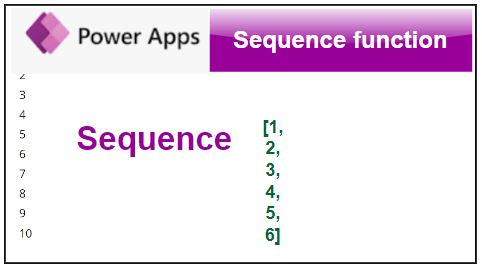The Sequence function in Power Apps generates a single column table of sequential numbers.

The name of the column returned by Sequence function is Value. It is limited to 50,000 records.

Syntax:

`Sequence( Records [, Start [, Step ] ] )`

Records: It is required, this is integer value to create the number of records that we want to generate and it must be in the range 0 to 50,000.

Start: It is optional, this is the starting number for the sequence. The default value for this is 1, if not provided.

Step: It is optional, this is an increment for each successive number in the sequence. The default value for Step is 1, if not provided. It also allows negative value, which can be negative to count down from the Start.

Let’s use a sequence function to generate a sequential numbers.

#### Generate sequential number starting from 1 to 10

Let’s add one Data table to show sequential numbers, go to Insert tab then search for Data table and click to add and named it as dt_sequence.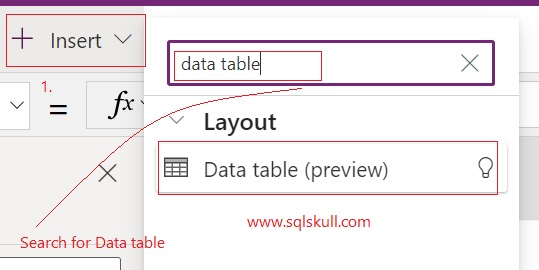Now, go to Item property of Data table and write below code.

`Sequence(10)`

Lets understand the above code, the value provided to first argument is 10, means sequence function will return 10 records. It will take default value for second argument (Start) and third argument (Step) which is 1. Means, sequence function will generates sequential numbers starting from value 1 and incrementing by 1 to previous value.

You can quickly see the output, just select the code in formula bar and you can see the values returned by Sequence function.

It returns single column table of sequential numbers from 1 to 10, and the name of column is Value.You can also, show the values returned by Sequence function in data table.

Just, go to the property of Data table, click on Edit field link and, then click on Add field and select the Value.

After that, click on Add button. Now, you can see the sequential numbers are displayed in data table.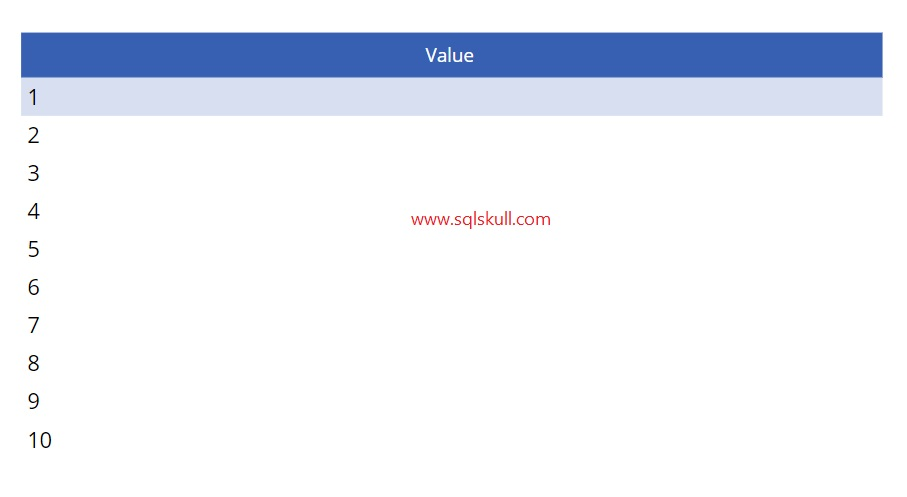#### Generate numbers starting from 1 to 10, incrementing by 2

Let’s modify the above code as shown below.

```Sequence(
10,
1,
2
)```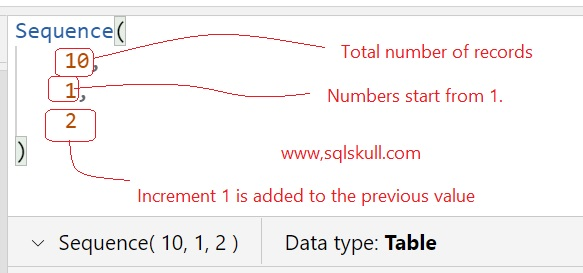Let’s understand the above code, the value provided to the first argument is 10, means we want it to return total 10 records.

The value provided to second argument is 1, means numbers will be started from 1.

The value provided to third argument is 2, means increment 1 is added to the previous value in sequence.

You can see it returns total 10 records starting from 1 and incrementing by 2.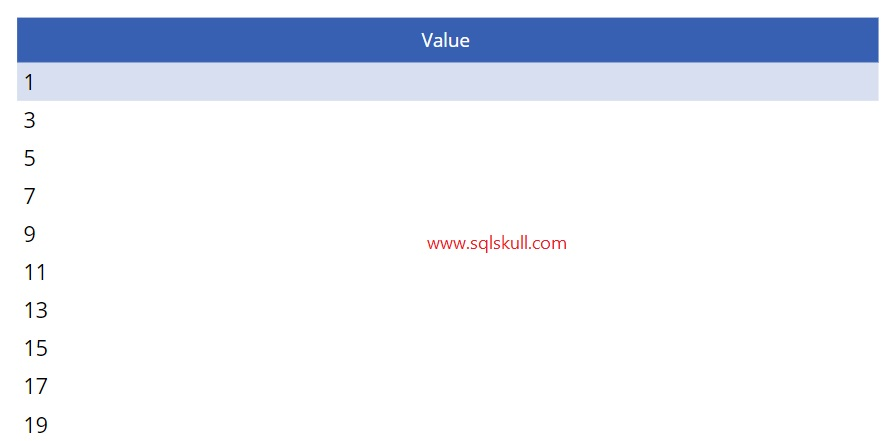#### Generate numbers starting from 10 to 1, incrementing by -1

Let’s modify the code as shown below.

```Sequence(
10,
10,
-1
)```Let’s understand the above code, the value provided to the first argument is 10, means it will return total 10 records.

The value provided to second argument is 10, means numbers will be started from 10.

The value provided to third argument is -1, means increment -1 is added to the previous value in sequence.

Let’s see, the output in data table, and you can see sequential values are started from 10 by incrementing -1.#### Using Sequence function with ForAll function

You can also use Sequence function with ForAll function, in case if you want to iterate any formula at specific number of times.

Generate random numbers using Sequence and ForAll function

Following code generate 5 random numbers

```ForAll(
Sequence(5),
Rand()
)```

You can see, it returns a table of 5 random numbers.Let’s understand the above code, we have used Sequence function to generate 5 sequential numbers and on the top of that used ForAll function to iterate the rand() function for 5 times to generate random number.